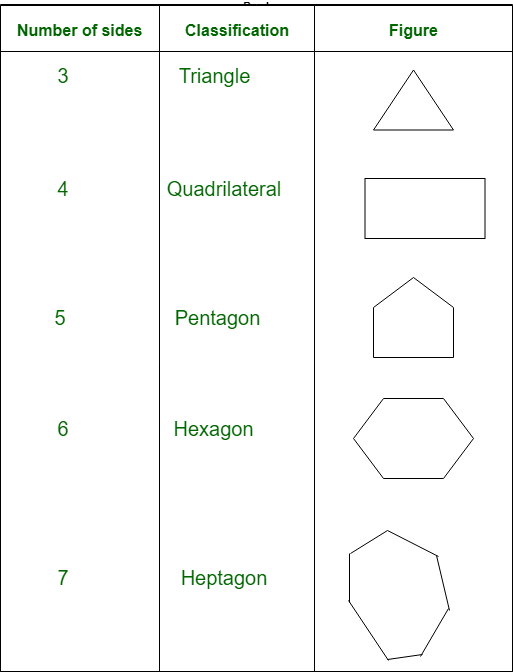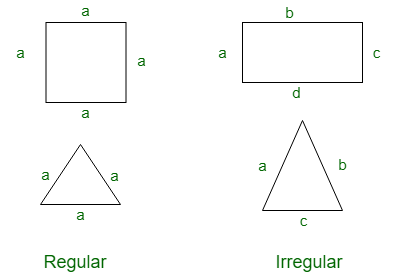GeeksforGeeks App
Open AppBrowser
Continue

# GRE Geometry | Polygons

Polygons:
The word polygon is derived from the Greek word polugonon, here poly means many and gons means angles. Polygons can be defined as a closed curve made up of line segments. Each line segment will become sides of the polygon.

Classification of polygons:
On the basis of the number of sides polygons are classified as follows:For n sides, we can call it n-gon. From here we can observe that the triangle is a polygon with least number of sides.

Concave and Convex polygon:
Convex polygons are the polygons whose all diagonal lie inside the figure and Concave polygons are those whose any diagonal lie outside the figure.Regular and Irregular polygon:
Regular polygons are the polygons having all sides of equal length and Irregular polygons are the polygons having different side length.Note:

• For a polygon, Exterior angle + Interior angle = 360°
• The sum of all exterior angles of polygon is 360°
• In a regular polygon all interior angles are equal.
• In a regular polygon all exterior angles are also equal.
• For a regular polygon, Number of sides = 360/exterior angle.
• Sum of all interior angles of a polygon is given by (n-2)*180, where ‘n’ is the number of sides in the polygon.
• Area of a polygon means area enclosed by the polygon and Perimeter of a polygon is sum of all the side length.

Examples:

• Example-1: What is the sum of all interior angles of heptagon ?
Solution:

```Since it is a Heptagon, number of sides (n) = 7
Sum of all interior angles = (n-1)*180 ```

So, for heptagon sum of interior angles = (7-2)*180 = 900

• Example-2: Calculate the number of side in a regular polygon having exterior angle 45° ?
Solution:

```Number of sides = 360/exterior angle
Since exterior angle is 45°
number of sides = 360/45 = 8 ```
• Example-3: What will be the value of an interior angle for a regular hexagon?
Solution:

```Since it is a hexagon, number of sides (n) = 6
exterior angle = 360°/6 = 60
we know that, interior angle + exterior angle = 180°
interior angle + 60° = 180°
interior angle = 180° - 60°
interior angle = 120 ° ```
• Example-4: Exterior angle of a regular polygon is 8°.
```(a) find the number of sides in the polygon?
(b) find the interior angle of the polygon? ```

Solution:

```(a) Number of sides = 360°/8°= 45
(b) interior angle = 180°- 8° = 172 ```
My Personal Notes arrow_drop_up